# Monte Carlo Simulation: Definition, Example, Code

Years ago, I had made it to the ﬁnal round in an interview for a Senior Delta One/Quantitative Futures position at an HFT ﬁrm (unnamed for privacy). Things were going well, I had answered two out of three of those ridiculous questions that are only applicable in Subsaharan Africa or Finance interviews (Like how to get 5 gallons from a 6 and 4-gallon jug); I was feeling good. They asked me about my optimization process — a layup compared to most — and I went through my process and ended with Monte Carlo Simulation, where their Head of Quant asked me How I run Monte Carlo Simulations, and what parameters I use.

The easy answer is “I run it in Multicharts”, I click Monte Carlo — but I decided to try to explain my Python code. I got so wrapped up in it, by the end of it I had lost my place and forgotten what Monte Carlo is really doing at its core. What should have been a home run became a sloppy drawn out mess of an answer while missing the key points. I essentially explained the worlds most confusing backtest/parameter optimization, and blanked on what was unique by the time I got there in my explanation. I want to make a point to emphasize that there’s a lot more to Monte Carlo’s than colourful line plots.

Luckily I did later realize what I was grasping for — and I used my favourite analogy; If a backtest is a Ladder, Monte Carlo is randomly rearranging the rungs on that ladder, and determining the likelihood of possible outcomes. THAT is an answer — if only it were my ﬁrst answer. Needless to say, I wasn’t offered this job, but it taught me an important lesson — knowing what your models and code are doing is just as important as being able to write them.

After reading this article, I will ensure you don’t fall victim to coding yourself into a corner with a model like Monte Carlo. First of all, let me clarify that there are a few different types of Monte Carlo optimizations — they are not all created equally. First of all, there is entirely random Monte Carlo’s, Random within a Normal Distribution Monte Carlo’s, and simple Random Trade Order. Random can be further sectioned into with or without replacement, but I will leave it at these three types

—which should make more sense to you as we continue. I will primarily focus on entirely (pseudo) Random Monte Carlo’s, as I ﬁnd them to be the most useful / least prone to error (for more info on limitations of Normal Distributions, I encourage you to read the Incerto series by Nassim Nicholas Taleb).

Many of you have either heard of or extensively used Monte Carlo methods of optimization or simulation — it can be an invaluable tool in measuring the unpredictable. They are not only useful in optimization problems but great for forecasting things like Max DD, or complex scenarios like the probability of your savings being sufﬁcient for retirement expenses. I primarily use them for two key parts of development; Portfolio Selection/Optimization, and System/ Portfolio Stress Testing. Before we dig into applications, let me explain what Monte Carlo simulations are.

Monte Carlo (MC) simulations are models used to model the probability of complex events by compiling thousands - millions of various outcomes with a pre-determined ‘random’ (changing) variable. Essentially you run 10k iterations with random values for a speciﬁc variable, in hopes of ﬁnding an optimum value or determining a range of possible outcomes — i.e. using randomness to solve a complex problem. A simple example is modelling the Maximum Sharpe Ratio of a Portfolio, based on ‘random’ security weights — so you have a Portfolio comprised of AAPL, AMZN, AMD, & ADBE and you want to determine the ideal weighting of these securities to maximize Sharpe.

The other more common scenario is using Monte Carlo simulations to determine the probability of outcomes — for example % Risk of Ruin with a portfolio, given its return characteristics (Mean, Std), and initial balance. This is where Monte Carlo simulations have applications in virtually every ﬁeld from Finance and Engineering to Logistics or Social Sciences. Many common metrics such as VaR and CVaR (Conditional Value at Risk) are derived at their core from Monte Carlo simulations, and have proven to be a valuable tool in a Quant’s toolkit.

The most important thing to take away from this is that Monte Carlo Sims are endlessly ﬂexible

—if there’s ever a problem that you need to solve that you cannot ﬁgure out, chances are Monte Carlo simulations can be used to get you pretty close to correct. Recently I was trying to ﬁnd a way to optimize a complex strategy that utilizes spreads of spreads in Futures markets, and this optimization algorithm was killing me. I decided that I could set the spread ratio as a random variable, and run it as a Monte Carlo simulation and at least get in the right direction — in 5 minutes & 100k iterations I had a simple 15 line solution to a problem that had taken me maybe 350 lines of Python when I initially tried to use a minimization function. This is the adjustable wrench of your toolbox.

Let’s dive in, and I’m going to over comment this code so it could not be clearer what’s doing what. First off, we need your security returns. This can be pulled from system performance (Like my example) or pulled with quantrautil using simple price data. The DF should be Securities Tickers as columns, with date rows and daily return values. Technically, it should be log-returns so I’ve included that calculation — but the difference is typically immaterial.

Begin by initializing the arrays for saving the performance values from the Monte Carlo runs, and set up the Monte Carlo simulations loop, deﬁning the # of runs as high as your PC will allow (start with 1k or so, scale-up). The important thing to remember is the weights, this is the Monte Carlo simulations magic, as this will select a new value each time and provide the power to the optimization, making each run unique. Also notice how each value your saving (ret, vol, Sharpe) is really an array being saved with indexing per each run — so run 0 begins, randomizes weights, and calculates the return, saving it to index 0 in ret arr, and then vol saves in 0 index, and ﬁnally SR. Once you’ve looped through, you run an argmax() function in the SR arr (or whatever value your maximizing), which will give you a weight, 477 in my example. This mean run 477 gave you the highest SR value, and that is your ideal weight! You can ﬁnd the optimal values in all weights, indexing with your max run number

(I also included a helper function to save the weights and tickers into a DF, and Pkl it for reference).

#Normalize returns--Nat Log -- Technically, it uses log returns, but the difference is immaterial.
#log_ret = np.log(ret/ret.shift(1)) #Calc for log returns, if official is important (This is instead of pct_change())
log_ret = pct
ret = log_ret
#Create Temporary (Random) weights
weights = np.array(np.random.random(15)) #USE NUM SECURITIESS

#Rebalance w/ constraints (CANNOT BE > 1)
weights = weights/np.sum(weights)
print(weights)

#DEFINE ARRAYS FOR STORING METRICS
num_runs = 1000    #Kick this number up 10k-100k for better results
all_weights = np.zeros((num_runs,len(ret.columns)))
ret_arr = np.zeros(num_runs)
vol_arr = np.zeros(num_runs)
sharpe_arr = np.zeros(num_runs)

#Begin MC Loop
for run in range(num_runs):

#Weights
weights = np.array(np.random.random(15))  #CHG to number securities *** THIS is the key to MC, this random value creation for weights
#If you're looking for a challenge, try using a poisson, gamma or Student T dist!
weights = weights/np.sum(weights)

#Save weights (For reference Later)
all_weights[run,:] = weights

#Expected Ret (Record each runs return in ret_arr)
exp_ret = np.sum((log_ret.mean() * weights) * 252)
ret_arr[run] = np.sum( (log_ret.mean() * weights) * 252) #Time =  year

#Exp Vol: (Lets attempt some linear algebra w/out runtime error!)
exp_vol = np.sum((log_ret.std() * weights) * 252)
# Sqrt of dot product of Transposed weights X Cov of Log returns & weights--whew.
vol_arr[run] = np.sqrt(np.dot(weights.T,np.dot(log_ret.cov()*252,weights)))

#Sharpe
SR = exp_ret/exp_vol
sharpe_arr[run] = ret_arr[run]/vol_arr[run]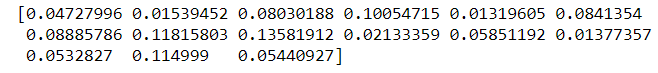If you’re a visual person like myself, you can plot it with a quick pyplot, using the retarr and volarr max values, and can select the max Sharpe to be highlighted as shown. That wasn’t so bad!

import matplotlib.pyplot as plt
'''Plot the Markowitz efficient frontier'''
plt.figure(figsize=(12,8))
plt.scatter(vol_arr, ret_arr, c=sharpe_arr, cmap='cividis')
plt.colorbar(label='Sharpe Ratio')
plt.xlabel('Volatility')
plt.ylabel('Return')
plt.title('Omni Efficient Frontier')
plt.scatter(max_sr_vol, max_sr_ret,c='red', s=50) # red dot
plt.show()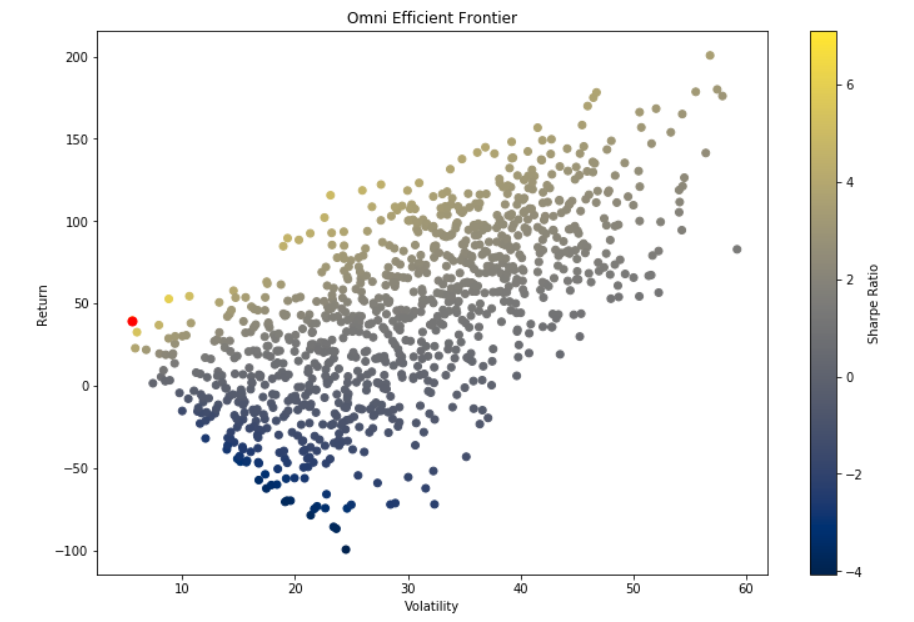Remember, you can make these return and volatility columns maximize anything you’d like — Correlation, Beta, anything. You can also randomize anything you’d like to optimize for within reason — you just need to ensure the logic works, and that it’s incorporated properly (Hint: I’ve taken entire strategies, and simply put a Monte Carlo simulations loop at the VERY end when calculating return, and added weights or thresholds in to be randomized and multiplied by the returns to optimize for them — it could even theoretically be randomized before the entries, just put a loop in there and randomize your entry characteristics.). My hope is to open you up a world of possibilities for Monte Carlo simulations to solve equations you never thought possible.

#sharpe_arr.argmax()
#MC_SR = sharpe_arr #Plug in to sharpe_arr10.094382

#find max SR per vol arr and SR array (for plotting)
max_sr_vol = vol_arr[sharpe_arr.argmax()]
max_sr_ret = ret_arr[sharpe_arr.argmax()] #85563 is max idx

#Save optimal weights, calc SR_annualized,
idx = sharpe_arr.argmax()
#all_weights[idx]
MC_SR = sharpe_arr[idx]
print(MC_SR)
SR_ann = np.sqrt((MC_SR))*12
SR_ann

print('Max Sharpe:',MC_SR)
print('Max Annualized Sharpe',SR_ann)
print('max sr ret:',max_sr_ret)
#print('Max sr vol:',max_sr_vol)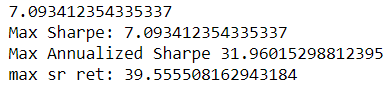My next example is a more common Monte Carlo simulations method, using Portfolio characteristics to predict expected returns, variance and worst-case scenarios. I’ll use the same data in my example, and plot them out for visualization. Don’t worry, this one is MUCH simpler.

This example simply requires you to pull the mean daily (log) return and the daily standard deviation of your system/portfolio. Once you plug those values in, you have everything you need, just plug in a number of iterations in the range(), and ensure your plot is INSIDE the loop, with the .show() outside.

import numpy as np
import numpy.random as nrand
import matplotlib.pyplot as plt
import math

#Define vars
S = 1000000
T = 252
mu = 1.92246
vol = .86123  #Between .76 and .86

result = []
for i in range(1000):
daily_returns = np.random.normal(mu/T,vol/math.sqrt(T),T)+1

price_list = [S]

for x in daily_returns:
price_list.append(price_list[-1]*x)
result.append(price_list[-1]) #appending each runs end value --to calculate the mean return

plt.plot(price_list)#This is key, KEEP THIS IN LOOP,votherwise it will plot one iteration/return path.
plt.title('Daily MC')
plt.show()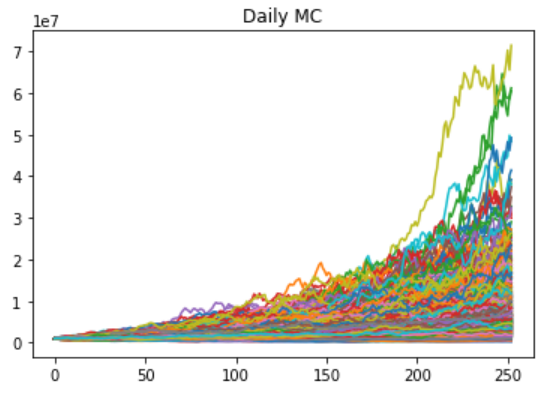This chart, while somewhat pretty, is not very useful; my preferred method of utilizing this is to plot it as a distribution and take various metrics of the universe of portfolio runs. Keep in mind, this is simply using your daily mean, and standard deviation to run 1000’s of years of 1 yr (T value) performance trajectories. I used a normal distribution for randomness factor here to make the histogram cleaner, but you can use any random distribution or entirely random value/sample you would like here! Play around with various models, and see how they vary.

res = [i/S*100 for i in result]
plt.title('MC: Annual Returns %')
plt.xlabel('Return (%)')
plt.ylabel('Distribution')
plt.hist(res,bins=100)
plt.show()

print('Mean return %:',np.mean(res))
print('Median return %:',np.median(res))
print('Min return %:',np.min(res))
print('Max return %:',np.max(res))
#print('Mode:',stats.mode(result))
print('Stdev %', stdev(res))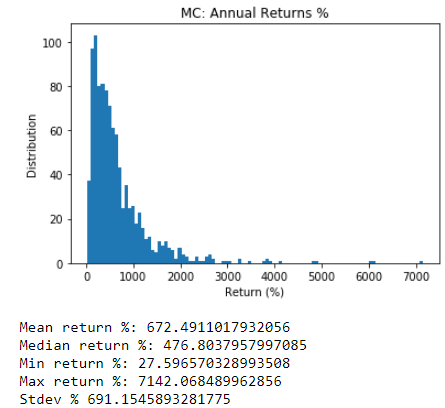I like to calculate various percentiles and track minimums along with various common metrics. The histogram provides a much clearer picture — in absolute terms, it can just seem large so I like to divide it by initial account value to make it percent values instead. This should be straightforward, aside from maybe the list comp, which simply is takings each runs result as a percentage of the initial account value.

from scipy import stats
from statistics import stdev

print('Mean:',np.mean(result))
print('Mean Ret:',np.mean(result)/S*100)
print('Median:',np.median(result))
print('Median Ret:',np.median(result)/S*100)
print('Min:',np.min(result))
print('Min Ret:',np.min(result)/S*100)
print('Max:',np.max(result))
print('Max Ret:',np.max(result)/S*100)
#print('Mode:',stats.mode(result))
print('Stdev', stdev(result))
mc_mu = np.mean(result)
med = np.median(result)
mc_min = np.min(result)
mx = np.max(result)
std = stdev(result)

metrics = [mc_mu,med,mc_min,mx]
print('sharpe:',mu/vol)

print('5% Quantile',np.percentile(result,5))
print('5% Quantile %',np.percentile(result,5)/S*100)
print('95% Quantile',np.percentile(result,95))
print('95% Quantile %',np.percentile(result,95)/S*100)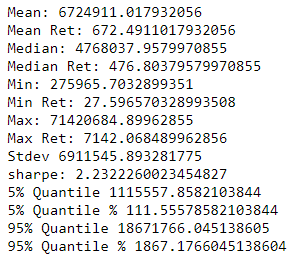So there we have it — Monte Carlo Simulations are one of the most ﬂexible models we have at our disposal, becoming comfortable with the inner workings of these models can make all the difference in optimizing complex problems. I hope you’ve also learned not to answer a Monte Carlo interview question with a complex answer that misses the point, dig into the basic moving parts as that’s where the magic really is in these models. Mastering Monte Carlo’s will provide you with the tools to solve otherwise insurmountable equations and untenable problems — or of course make really colourful line plots.